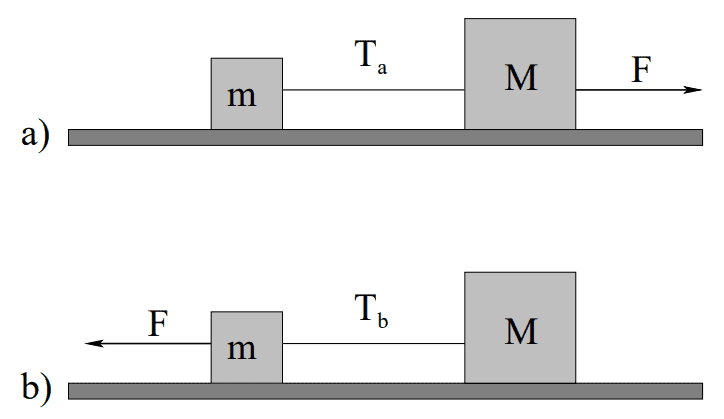# Friction, mass and tension

## Homework Statement

In the figure above a block of mass m is connected to a block of mass M > m by a string. Both blocks sit on a smooth surface with a coefficient of kinetic friction µk between either block and the surface. In figure a), a force of magnitude F (large enough to cause both blocks to slide) is exerted on block M to pull the system to the right. In figure b), a force of (the same) magnitude F is exerted on block m to pull the system to the left. Circle the true statement:
a) The tension Ta > Tb.
b) The tension Ta < Tb.
c) The tension Ta = Tb.
d) There is not enough information to determine the relative tension in the two cases.

known data:
we know that both blocks have the same coefficient of friction and that their masses are different.

ΣF= ma
μk= Fk*N

## The Attempt at a Solution

I drew first the FBD of both block in each situation and wrote the equation. for the first situation (a)
Block M has this equation:
ΣF= ma
F-Ta-Fk=Ma
Ta=F-Fk-Ma
Ta=F-μkMg-Ma
while block m I ended up with:
Ta=ma+μkmg

and for the second situation:
block M equation:
Tb= Ma+μkMg
and block m:
Tb=F-μkmg-ma

so I said since larger mass has larger KInetic Friction Fk, compared to the small one, and because of comparing the Ta equation of M and the Tb equation of M then Tb I guess is bigger.
I don't know if Iam right or not.

#### Attachments

•berkeman

Several things. First, the problem doesn’t explicitly state it, but you should evaluate this at constant velocity, ie a=0. Second, your approach is more complicated than it needs to be. You wind up with answers in “F” which you don’t know. You can figure out F but why bother? Look at figure a). What is the tension if m is 0? Clearly the tension is all about block m, and you can evaluate it considering only the forces on m. Similarly in case b you need only evaluate the forces on M.

•SakuRERE
Several things. First, the problem doesn’t explicitly state it, but you should evaluate this at constant velocity, ie a=0. Second, your approach is more complicated than it needs to be. You wind up with answers in “F” which you don’t know. You can figure out F but why bother? Look at figure a). What is the tension if m is 0? Clearly the tension is all about block m, and you can evaluate it considering only the forces on m. Similarly in case b you need only evaluate the forces on M.

I Knew i was complicating things more than how it should be.
So just to make sure, It's all about that mass being dragged by the rope, and since at the second situation The bigger mass and one having bigger fk then considering the system having the same acceleration :
Tb of M= Ma+fk
Ta of m= ma+fk
so Tb>Ta
thanks again

Tb of M= Ma+fk
Ta of m= ma+fk
so Tb>Ta

Like I said,I would take a to be 0, so in what you wrote above it wouldn’t be clear which is larger. However you wrote “fk” in both, which I presume means the force from sliding friction. Is that the same in the two cases? What is the magnitude of the frictional force in terms of the mass?

•SakuRERE
Like I said,I would take a to be 0, so in what you wrote above it wouldn’t be clear which is larger. However you wrote “fk” in both, which I presume means the force from sliding friction. Is that the same in the two cases? What is the magnitude of the frictional force in terms of the mass?
you're right, I must have written Fk of M and Fk of m,
since Fk= μk*Fn (Fn is the normal force) and it's mentioned that both blocks have the same μk
so we know both blocks have different masses and then they have different Fn because of Fn=Fg=mg (weight)
and I think there is no problem considering the acceleration a= constant value rather than a=zero since all other variables we know how they are different in each block.

you're right, I must have written Fk of M and Fk of m,
since Fk= μk*Fn (Fn is the normal force) and it's mentioned that both blocks have the same μk
so we know both blocks have different masses and then they have different Fn because of Fn=Fg=mg (weight)
and I think there is no problem considering the acceleration a= constant value rather than a=zero since all other variables we know how they are different in each block.

True. Your answer is more general and you see both terms are proportional to mass. However I wanted you to show that the second term is proportional to mass as I believe that is the point of the question (the first term having nothing to do with friction)

•SakuRERE
True. Your answer is more general and you see both terms are proportional to mass. However I wanted you to show that the second term is proportional to mass as I believe that is the point of the question (the first term having nothing to do with friction)
I do get what you mean, I have always been afraid of coming with assumptions from my mind. But at the end, you are an expert, and I see why you suggest studying the system when a=0 because we already have the mass (which is the only important thing in the first term) in the second term as a weight. I wish I got your idea right.
thanks again